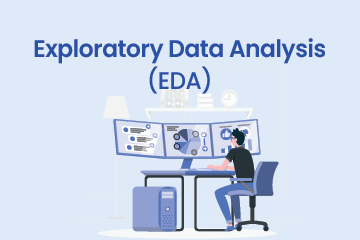Home / Blog / Data Science / Density Based Special Clustering | DBSCAN Algorithm

# Density Based Special Clustering | DBSCAN Algorithm

• June 13, 2023
• 2717
• 65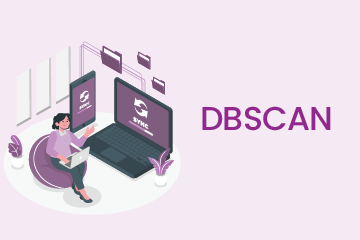### Meet the Author : Mr.Ritendu

Bharani Kumar Depuru is a well known IT personality from Hyderabad. He is the Founder and Director of Innodatatics Pvt Ltd and 360DigiTMG. Bharani Kumar is an IIT and ISB alumni with more than 17 years of experience, he held prominent positions in the IT elites like HSBC, ITC Infotech, Infosys, and Deloitte. He is a prevalent IT consultant specializing in Industrial Revolution 4.0 implementation, Data Analytics practice setup, Artificial Intelligence, Big Data Analytics, Industrial IoT, Business Intelligence and Business Management. Bharani Kumar is also the chief trainer at 360DigiTMG with more than Ten years of experience and has been making the IT transition journey easy for his students. 360DigiTMG is at the forefront of delivering quality education, thereby bridging the gap between academia and industry.

Table of Content

## Introduction:

Density Based Special Clustering of Application with Noise is known as DBSCAN. Oh my god big full form. Don’t worry, this is not difficult in its own. It’s very simple, I will make you understand what DBSCAN is. Basically, this is a clustering algorithm in machine learning.

## Why DBSCAN?

Well, we have k means and hierarchical clustering apart from DBSCAN. Then your question might be, why this DBSCAN. Basically, it can make a visualization of outliers. I hope you already know about outliers. So, it actually clusters the data according to their density, so which data is not near by and have outstanding value, it will not consider that value in the cluster. Also, another facility is we don’t have to mention the number of clusters, it will arrange the cluster according to the nearby points. All these explanations I will give in this whole blog, so don’t worry about these.

## DBSCAN Algorithm

To understand the algorithm, we have to understand the terminologies.

• Epsilon
• MinPts
• Core Points
• Border Points
• Noise

I will be coming to these points one by one.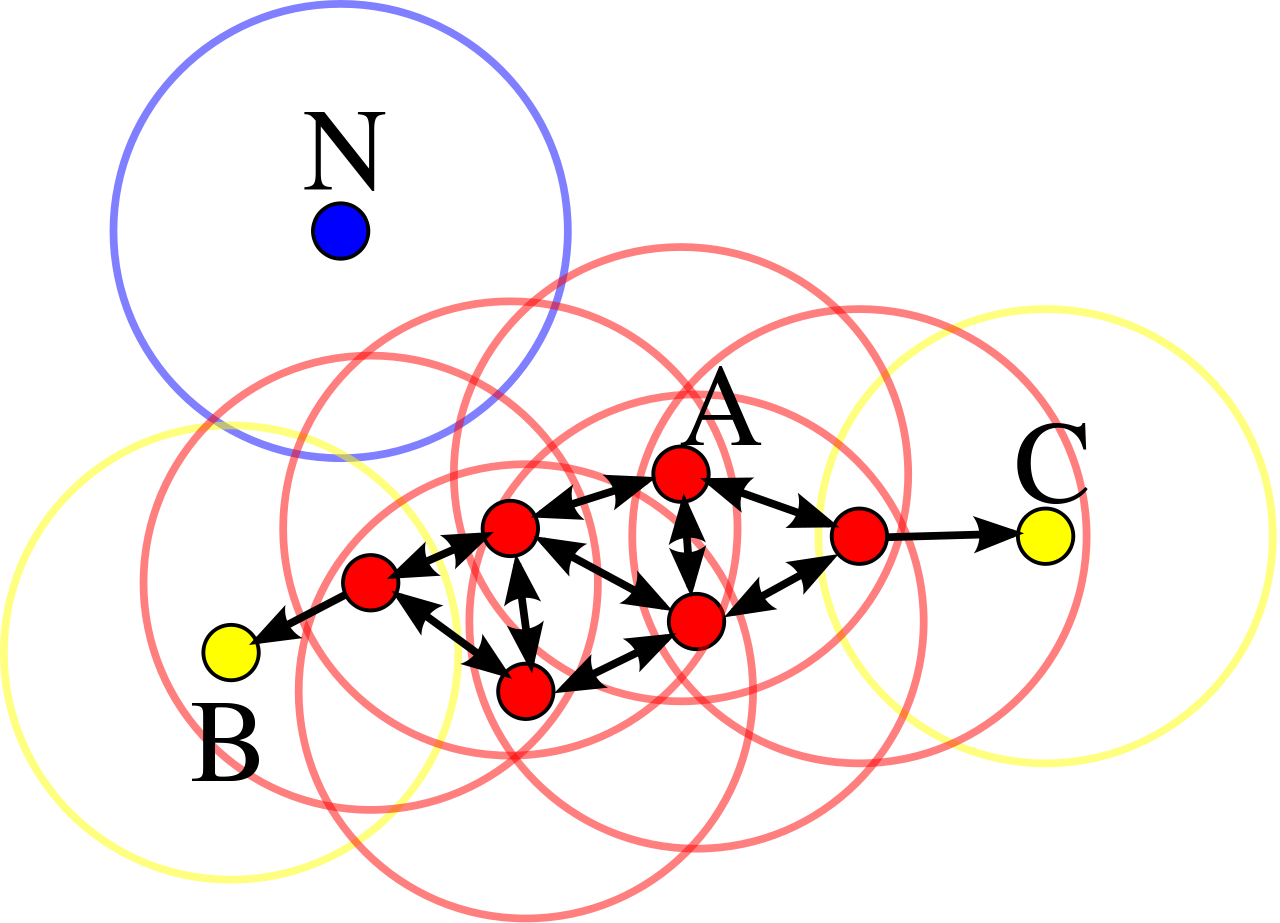Consider these datapoints. Now for example you are taking one point A. There you take one value, say 1.5 and make that as a radius from the center A. So, there you created one circle. And you said 3 minimum points I need inside that circle. From the diagram you can see that there are 4 points inside that circle which center is A. Now this A point will be called as a Core Point. This Radius will be called Epsilon and the 3 minimum points that you decided, will be called as MinPts.

This circle is not actually the whole cluster, but you are beginner stage to create a cluster based on DBSCAN.

So now what is the border points? Take B point from the diagram. Now again take 1.5 as epsilon and create one circle. Now in the previous case you also define one minimum point as 3. You can see that inside this circle you are having only one point. Which is not satisfying your condition of minimum point. But check that, which point is in the circle is a core point. Also, we can easily conclude that this core is the neighbor of the of B point. We will call this point as border point.

You may be totally confused that what is the border point? In simple words, take a radius of epsilon from B point and check whether the your MinPts satisfying or not. If not then check whether any core point inside the circle or not (check the neighbor basically). If so then this point is called as border point.

Now the one question remains that what is Noise? Noise is basically an outlier. Let me explain how I am saying this. Now from the diagram take the point N. Again, the same procedure, take the same epsilon value and create one circle. In that circle you can see there is no point. This point is actually Noise. Basically, what we are understanding from this situation is, N value is an outstanding one from the other datapoint from the dataset. So, we can easily say that this N is an outlier.

## Mathematics for DBSCAN: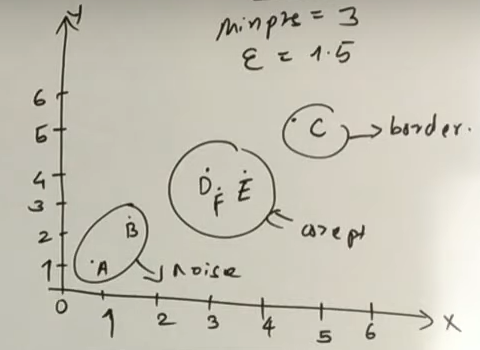For example, you have these datapoints. Here MinPts is 3 and epsilon is 1.5. Don’t get confused, these values are randomly taken. Now by seeing this diagram and reading the algorithm we can approximately say that,

D, E, F are core points

C is Border points,

A, B are Noises.

Now the point is how we can derive this mathematically. For that see the below image first.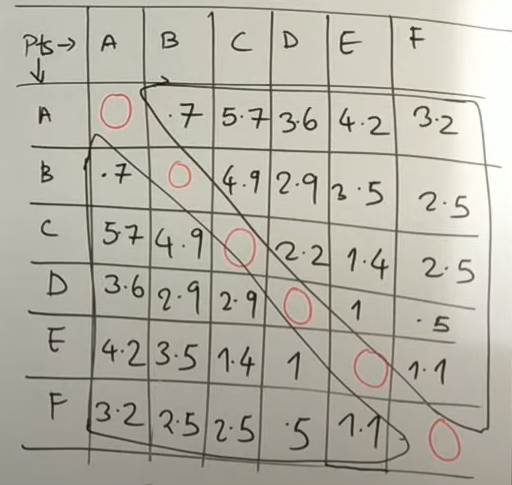This diagram is nothing but a distance metric for those points. I am assuming that you already know what is distance metric. Who is unaware of this? It is simply a list of all the distances between the points. Now consider the columns.

First for A, it’s not a core point because no distance is less than or equal to 1.5. only A to A is 0, other than that everything is greater than 1.5. So, assign it to Border/Noise point. [Note: I am not identifying the border or noise points as of now. Only considering either core point or border/noise point]

Now for B, again with the same logic we can say B is also not a core point because B to B is only 0, other than that everything is greater than 1.5. So, let’s assign it to Border/Noise point.

Now for C, again it’s not satisfying the MinPts condition. Because only 2 points have less than or equal to 1.5 value. C to C and C to E. So, assign it to Border/Noise point.

Now for D, you can see we have 3 points. D to D, D to E and D to F. These distances are less than 1.5. So, assign it to Core Point.

Now for E, here, you can see we have 4 points. E to C, E to D, E to E, E to F. These distances are less than 1.5. So, assign it to Core Point.

Lastly for F, here you can see we have 3 points. F to D, F to E, F to F. So assign it to Core Point.

Hurray! We identified the Core Points from the diagram. Now the last thing remaining is to divide which one is Border Point and which one is Noise Point.

So, consider C point first, there you can see C to E distance is less than 1.5, on top of that E is Core Point you identified. So, E core point is in the radius of C. So, from this scenario we can easily conclude that C is a Border Point.

Now check for A and B point, there you can see no point is in between the radius of A and B. Those are totally separate. So, now we can easily say that these points are Noise Points.

Congratulations, you successfully identified all the points from the dataset.

Now let’s come to real life scenario, there will not be these 5 to 6 data points. There may be thousand of data points. In that case also computer continuously calculate in this way for all the data points and create the clusters. See the below animated image to get a understanding.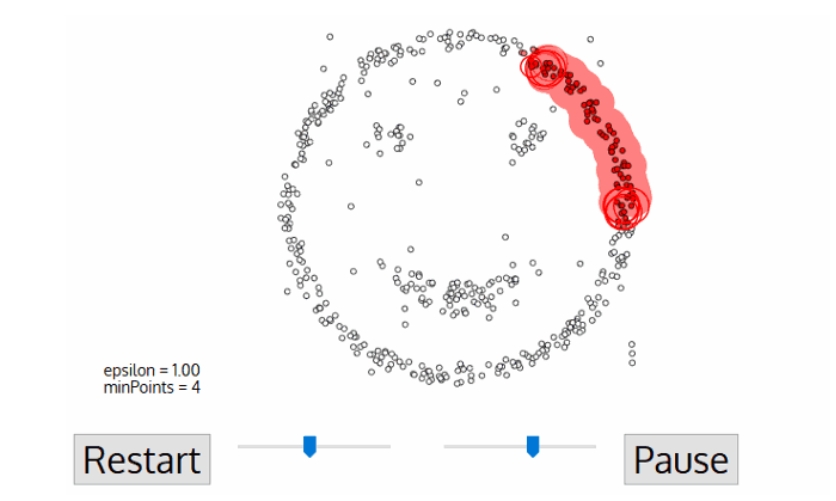## Conclusion:

So as a summary, you have to first take that epsilon and min pts. Then identify the core points, border points and noise points. By this procedure you will be able to form a cluster using density.

### Data Analyst Courses in Other Locations